GFG App
Open AppBrowser
Continue

# Lorenz Curve: Meaning, Construction, and Application

### What is Lorenz Curve?

The variability of a statistical series can be measured through different measures, Lorenz Curve is one of them. It is a Cumulative Percentage Curve and was first used by Max Lorenz. Generally, Lorenz Curves are used to measure the variability of the distribution of income and wealth. Hence, Lorenz Curve is the measure of the deviation of the actual distribution of a statistical series from the line of equal distribution. The extent of this deviation is known as Lorenz Coefficient. If the distance between the Lorenz Curve from the line of equal distribution is more, it means that there is more inequality or variability in the series, and vice-versa.

### Construction of Lorenz Curve

The steps involved in the construction of a Lorenz Curve are as follows:

Step 1: The first step is to convert the given series into a cumulative frequency series. Then it is assumed that the cumulative sum of the items (or mid-values of class intervals) is 100, and the different items of the series are converted into percentages of the cumulative sum. Similarly, it is assumed that the cumulative sum of the frequencies is 100, and different currencies are converted into percentages of the sum of the frequencies.

Step 2: In the second step, the cumulative frequencies are plotted on the X-axis of a graph, and the cumulative items are plotted on the Y-axis. To plot the values, we have to start from 0 to 100 on both axes.

Step 3: Now, draw a diagonal line joining the origin (0, 0) with the cumulative frequencies (100, 100). This diagonal line shows the equal distribution because of which it is known as the Equality Line or Line of Equal Distribution.

Step 4: Now, plot the actual data on the graph and obtain a curve joining the plotted points. This curve shows the actual distribution of the given statistical series.

The actual distribution curve is known as Lorenz Curve. If there is closeness in the Lorenz Curve to the Equal Distribution Line, it means that there is lesser variation in the distribution. However, if there is larger gap between the Lorenz Curve and the Equal Distribution Line, it means that there is greater variation in the distribution.

Besides, if two Lorenz Curves are drawn on the same graph paper, then the one which is further away from the equal distribution line shows greater variation.

#### Example 1:

Draw a Lorenz Curve of the data given below: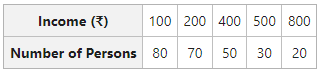#### Solution: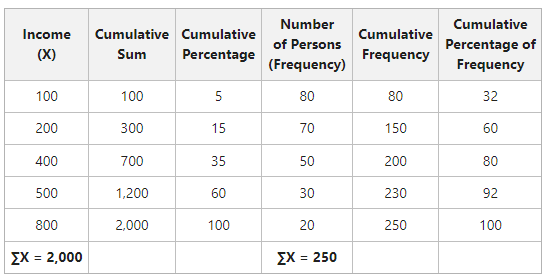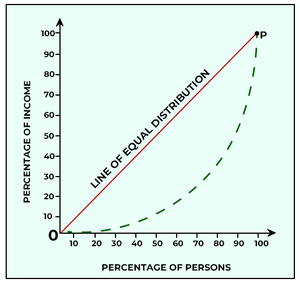#### Example 2:

Use Lorenz Curve to show inequality in wages in two different firms with the help of the following data: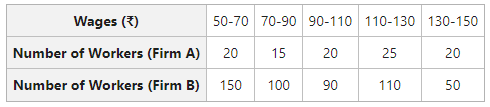#### Solution: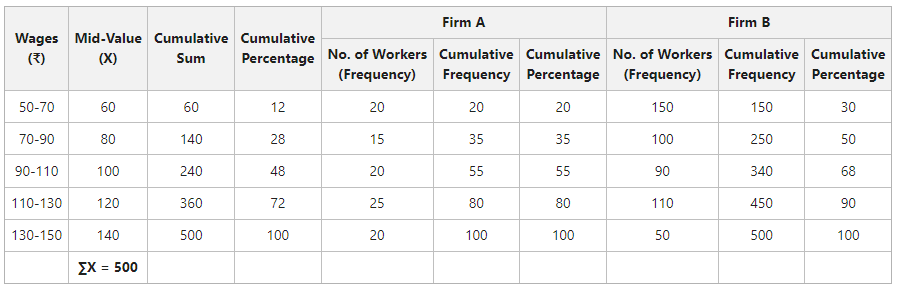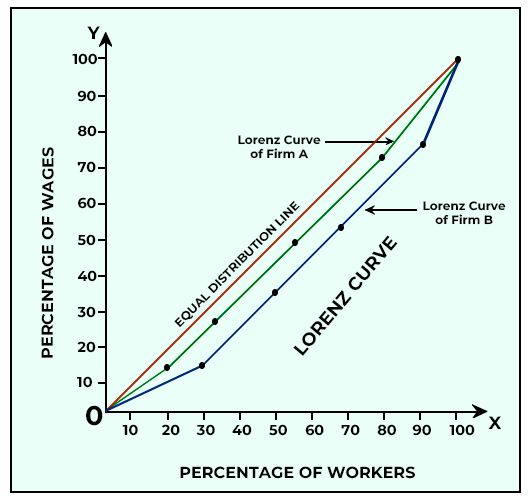Observation: In Firm A and B both, the distribution of wages is far from equal. The gap between the Lorenz Curve of Firm B and the Equal Distribution Line is more than for Firm A, which means that there is greater variation in the distribution of Firm B.

### Application of Lorenz Curve

A graphic measure of dispersion in a statistical series is known as Lorenz Curve. It provides the user with an immediate glimpse of the degree of variation in the given statistical distribution from its mean value; hence, is a simple measure. Prof. Lorenz first used this measure of dispersion for the measurement of economic inequality related to the distribution of income and wealth across different nations or different time periods for the same nation. Since then, the application of the Lorenz Curve has spread widely for the measurement of disparity of distribution related to various parameters distribution of wages and profits.

The parameters in which the Lorenz Curve is now applied for the measure of dispersion are as follows:

• Distribution of Income
• Distribution of Wages
• Distribution of Wealth
• Distribution of Profits
• Distribution of Production
• Distribution of Population

### Merits of Lorenz Curve

The merits of the Lorenz Curve are as follows:

1. It is attractive and gives a rough idea of the extent of dispersion.
2. Lorenz Curve makes it easy to compare two or more series.

### Demerits of Lorenz Curve

The demerits of Lorenz Curve are as follows:

1. With the help of the Lorenz Curve, one can only get a relative idea of the dispersion of the given distribution as compared with the line of equal distribution. Also, it does not provide the user with any numerical value of the variability for the given distribution.
2. It is difficult to draw Lorenz Curve.
My Personal Notes arrow_drop_up